# Diethylenetriamine, H2NCH2CH2NHCH2CH2NH2 (dien), is a tridentate ligand. Draw all the geometrical and optical isomers (f...

Diethylenetriamine, H2NCH2CH2NHCH2CH2NH2 (dien), is a tridentate ligand. Draw all the geometrical and optical isomers (four in total) that are possible for the complex [Co(dien)2]3+.

Solution:

Four isomers ( geometrical = fac, mer and optical = d and l) are given in attached sheet.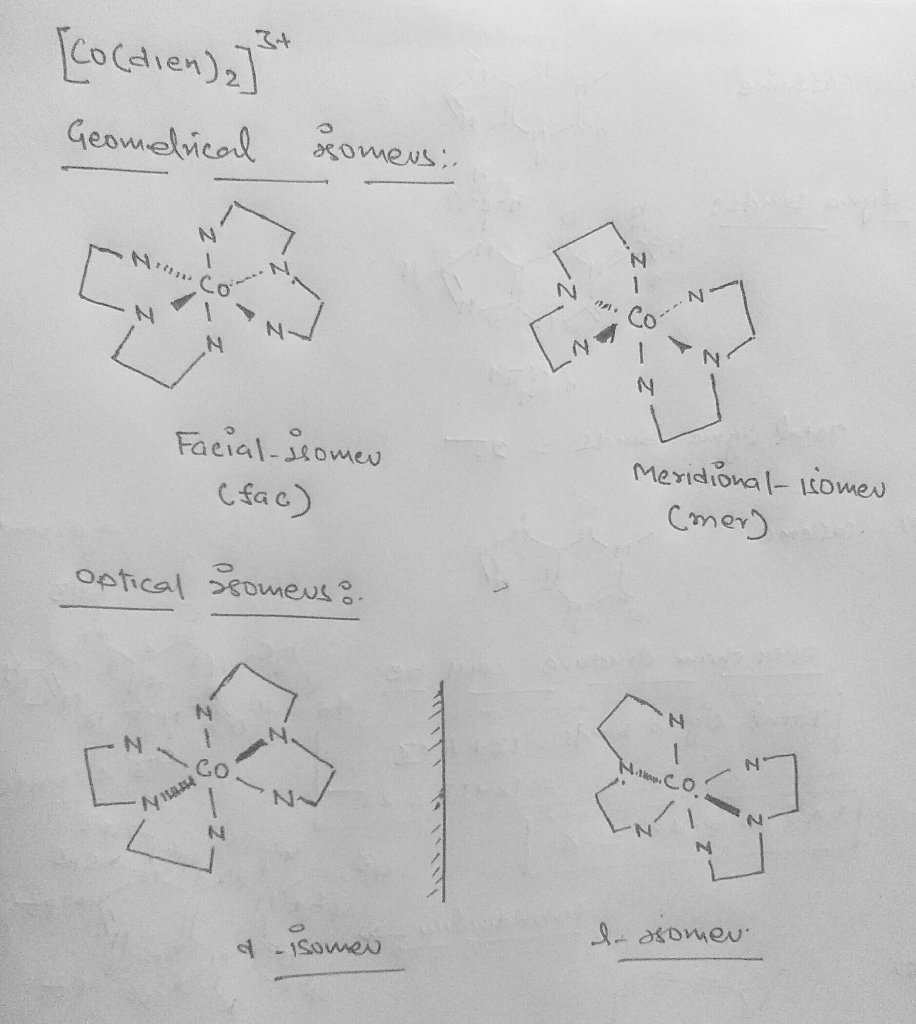##### Add Answer of: Diethylenetriamine, H2NCH2CH2NHCH2CH2NH2 (dien), is a tridentate ligand. Draw all the geometrical and optical isomers (f...
Similar Homework Help Questions
• ### 1.) Draw the possible isomers of [MnCl3(dien)] and name the complexes using the appropriate nomenclature procedures...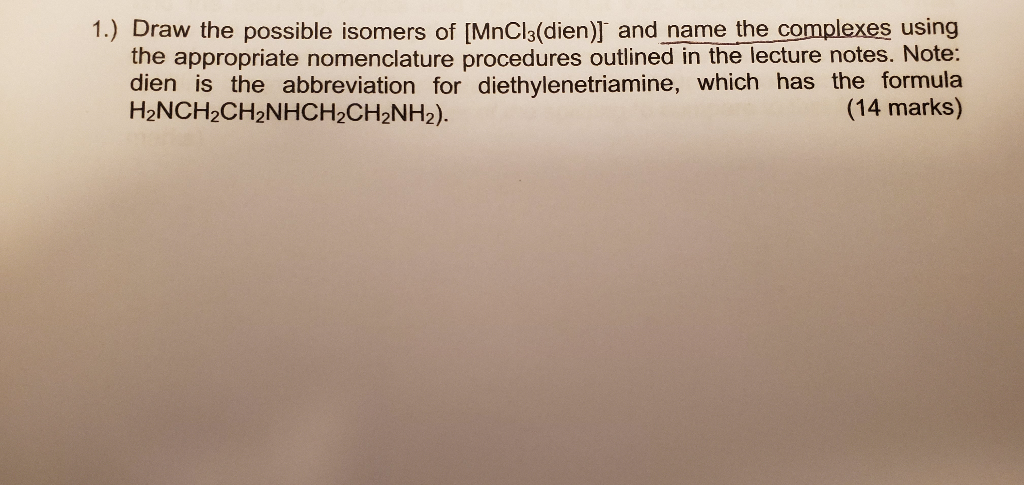1.) Draw the possible isomers of [MnCl3(dien)] and name the complexes using the appropriate nomenclature procedures outlined in the lecture notes. Note: dien is the abbreviation for diethylenetriamine, which has the formula H2NCH2CH2NHCH2CH2NH2). (14 marks)

• ### Name and draw the structures of the following complexes and complex ions and indicate any isomers...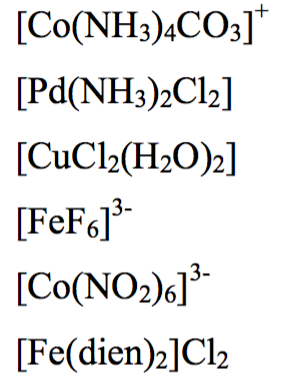Name and draw the structures of the following complexes and complex ions and indicate any isomers possible. NOTE: (dien) is diethylenetriamine [Co(NH3)4COl [Pd(NH3)2Cl2] [FeFo 3- [Fe(dien)2]Clb

• ### Octahedral complex

Consider the following complex, which has Co(III) in an octahedral geometry: [ Co(en)(dien)Cl]2+given; en= ethylenediammine=H2NCH2CH2NH2, which canbe abbreviated N-N dien = diethylenediammine=H2NCH2CH2NHCH2CH2NH2,which can be abbreviated N-N-NA) The ligand dien is a tridentate ligand.Explain what is meant by "tridentate" and why dien can act as atridentate ligand.B) Indicate if chiral or achiral (Think abouthow the dien ligand can bond; it is a flexible ligand)C) This complex could exist with either ahigh-spin or low-spin electron configuration. Provide thed-electron configurations expected in each...

• ### 5. How different stereoisomers (i.e. geometrical and optical isomers) of the complex are possible? many 3+...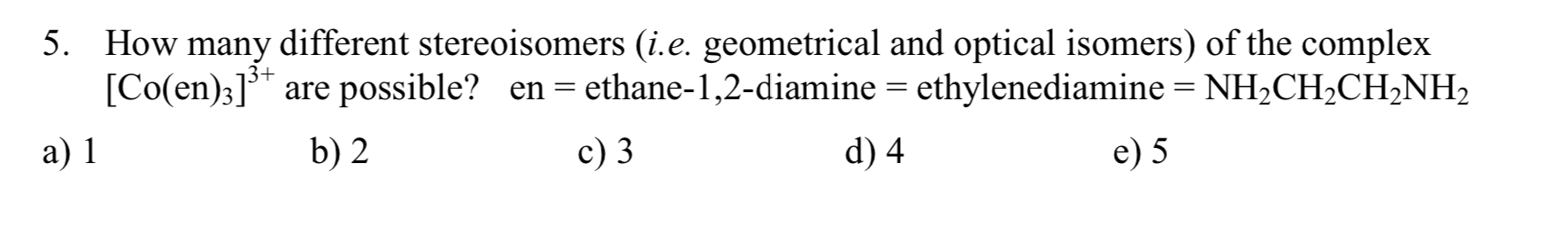5. How different stereoisomers (i.e. geometrical and optical isomers) of the complex are possible? many 3+ [Co(en)3] en = ethane-1,2-diamine ethylenediamine = NH2CH2CH2NH2 а) 1 b) 2 c) 3 d) 4 e) 5

Consider the following complex, which has Co(III) in an octahedral geometry: [Co(en)(dien)Cl]2+given: en= ethylenediammine =H2NCH2CH2NH2, which canbe abbreviated N-Ndien= diethylenediammine =H2NCH2CH2NHCH2CH2NH2,which can be abbreviated N-N-NDraw all the unique geometric isomers of this complex.For each one, indicate if it is chiral or achiral. (Think about howthe dien ligand can bond; it is aflexible ligand.)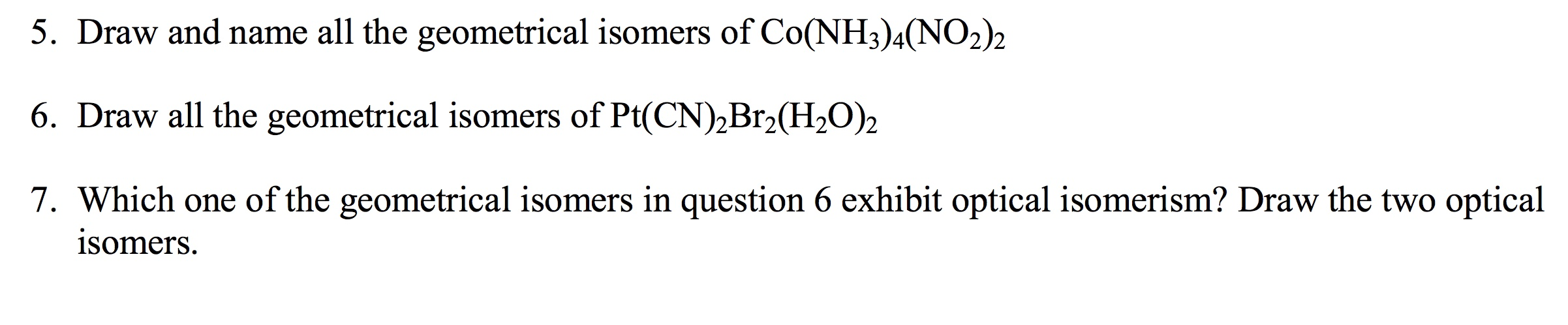These questions are linked, specifically 6 & 7. Please help & explain, especially #7. 5. Draw and name all the geometrical isomers of Co(NH3)4(NO2)2 6. Draw all the geometrical isomers of Pt(CN)2Br2(H20)2 7. Which one of the geometrical isomers in question 6 exhibit optical isomerism? Draw the two optical isomers.

• ### Draw geometrical isomers of each of the following complex ions. (a) [Co(H2O),(C,O,),]. (b) [Pt(NH,),12]2+ (c) [Ir(NHJ,C13]...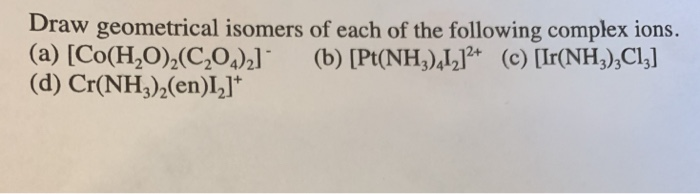Draw geometrical isomers of each of the following complex ions. (a) [Co(H2O),(C,O,),]. (b) [Pt(NH,),12]2+ (c) [Ir(NHJ,C13] (d) Cr(NH3)2(en)I2]t

• ### b) Sketch all possible geometrical isomers and then indicate which of these complexes would exhibit optica...b) Sketch all possible geometrical isomers and then indicate which of these complexes would exhibit optica activity. Also identify each complex by name a) [Fe(C,0.).cllion (C.0, oxalate ion), b) [PdCI,(PP) Q4. Assigne the point group of the following compounds (show all your work)

• ### 1. Name the following molecules: a) [PtBr(NH3)3]I3 b) [Ir(CO)2(OH)2]Cl c) K2[FeCl4(NO3)2] d) [((CH3)3N)3PdBr]Cl e) CoCl3H(dabco)2 2....

1. Name the following molecules: a) [PtBr(NH3)3]I3 b) [Ir(CO)2(OH)2]Cl c) K2[FeCl4(NO3)2] d) [((CH3)3N)3PdBr]Cl e) CoCl3H(dabco)2 2. Draw and give the point group for all the geometrical isomers of a molecule with the general formula: a) MA2B2 (square planar and tetrahedral) b) MA2B (“T”‐shaped and trigonal planar) c) MAB4 (trigonal bipyramidal and square pyramidal) d) MA2B3 (trigonal bipyramidal and square pyramidal) 4. Draw four distinct geometrical isomers of a metal complex of the type M(A‐CH2‐CH2‐B)2. Which of these is chiral? Give...

• ### Structural Geometry: Geometric and Optical Isomers

Draw structural formulas that show the geometry of the following including the geometric and optical isomers. (cis and trans) a.) [Co(C2O4)2(H2O)2]^-b.) [Ir(NH3)3CL3]c.) [Cr(en)(NH3)2I2]^+(Note: The numbers are subscripts, i couldn't type them like that. Just fyi)Thank you for anyone who will be helping me, its hard for me to comprehend the shape.

Need Online Homework Help?